CBSE Class 10 Sample Paper for 2021 Boards - Maths Basic

Class 10
Solutions of Sample Papers for Class 10 Boards

## Class X students of a secondary school in Krishnagar have been allotted a rectangular plot of a land for gardening activity. Saplings of Gulmohar are planted on the boundary at a distance of 1m from each other. There is a triangular grassy lawn in the plot as shown in the fig. The students are to sow seeds of flowering plants on the remaining area of the plot. Considering A as origin, answer question (i) to (v)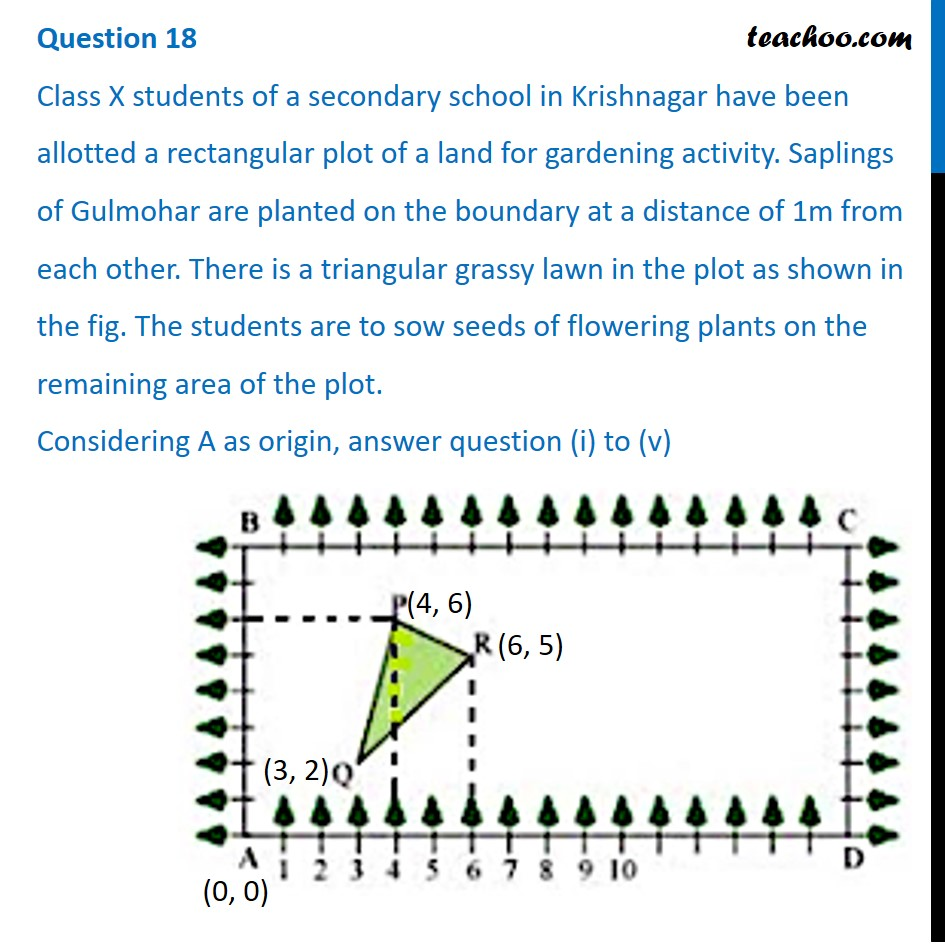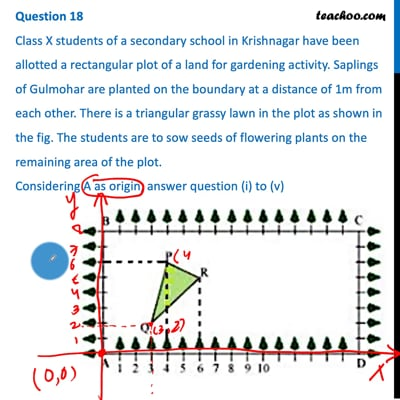This video is only available for Teachoo black users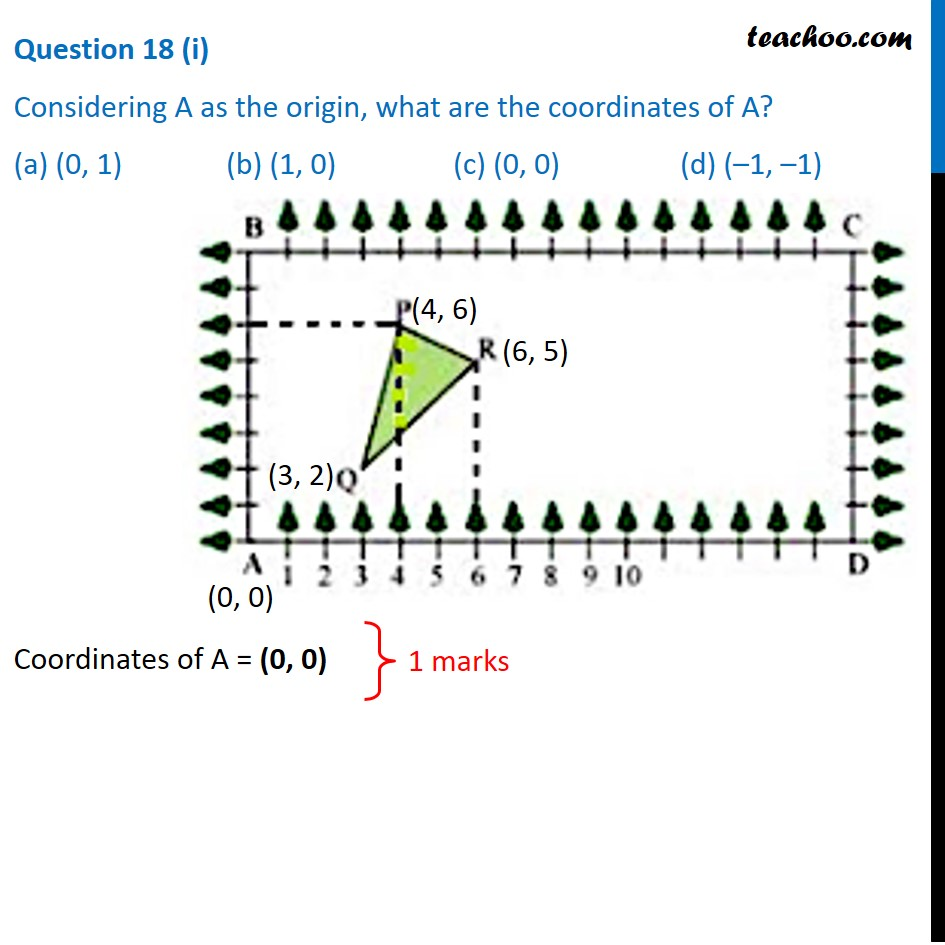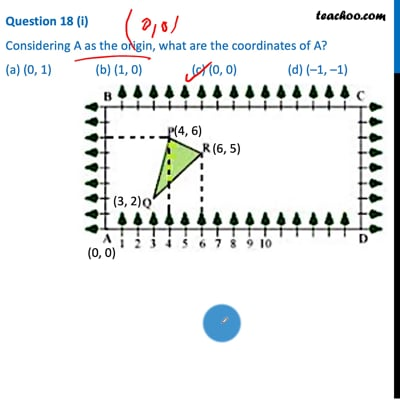This video is only available for Teachoo black users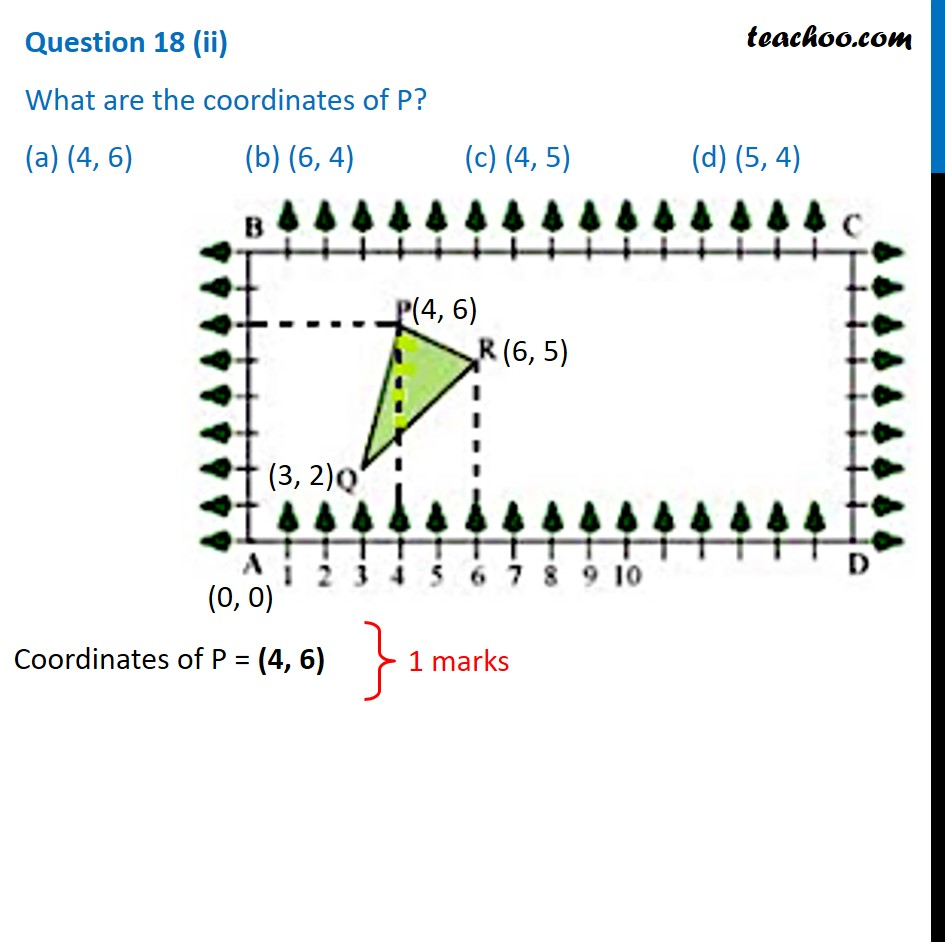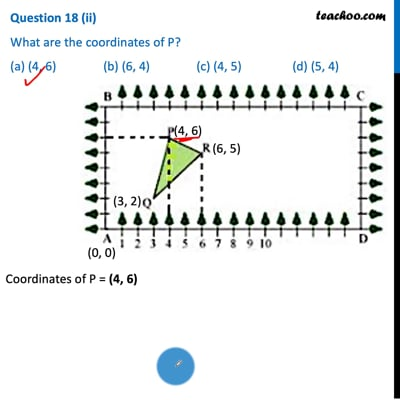This video is only available for Teachoo black users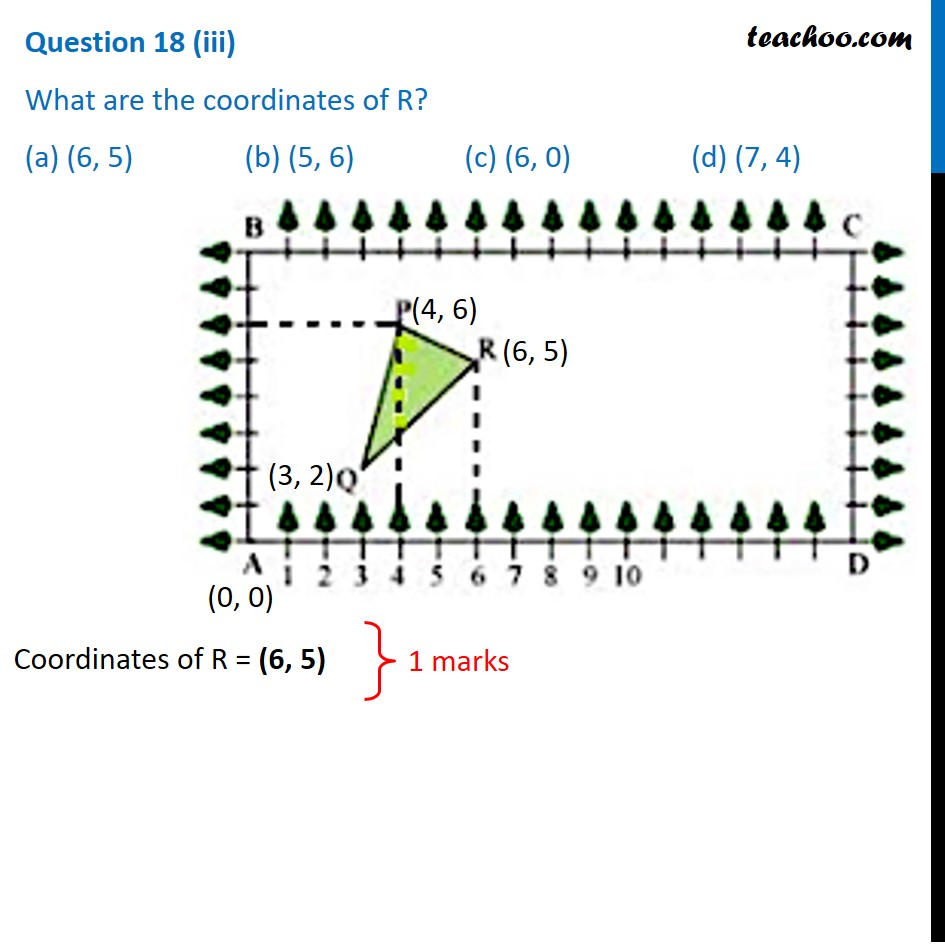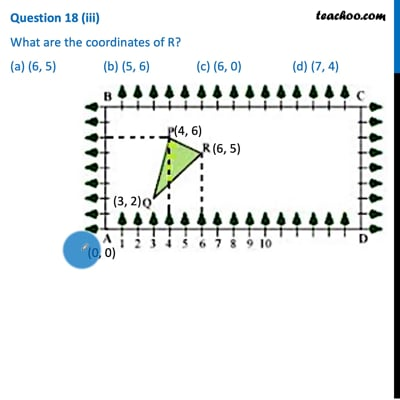This video is only available for Teachoo black users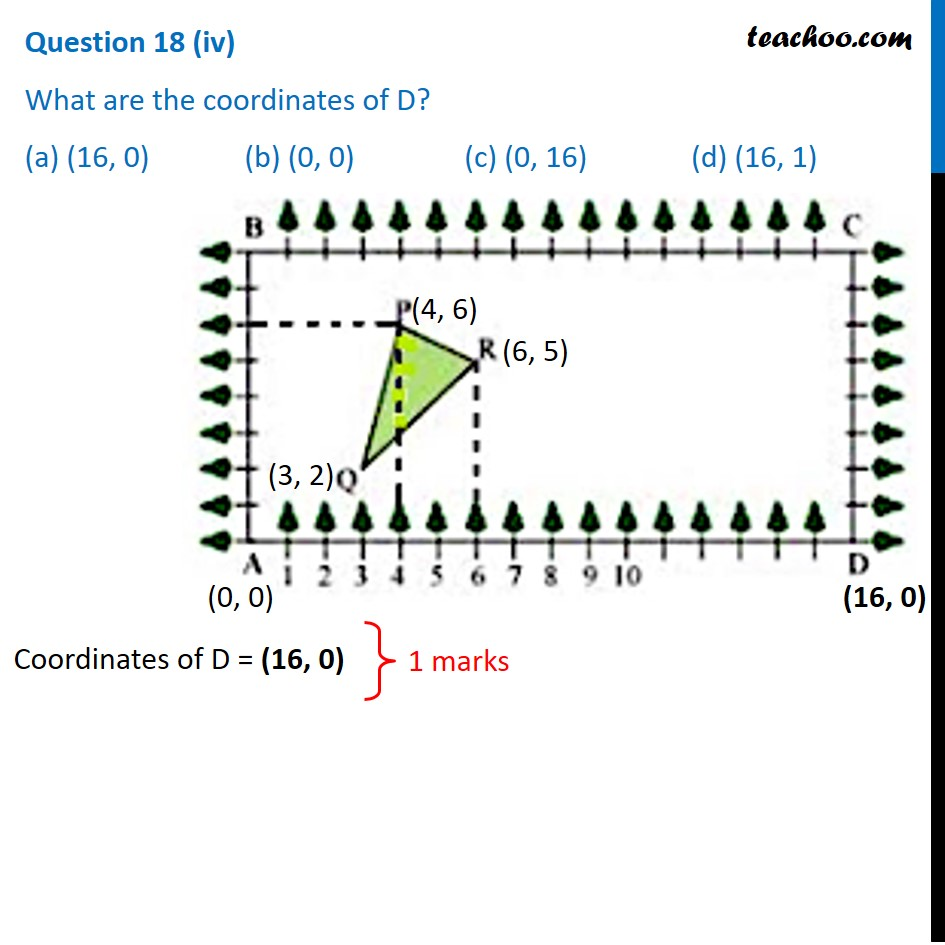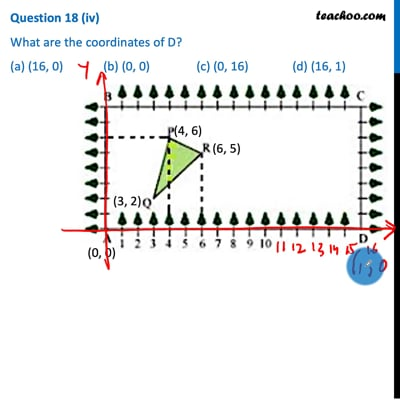This video is only available for Teachoo black users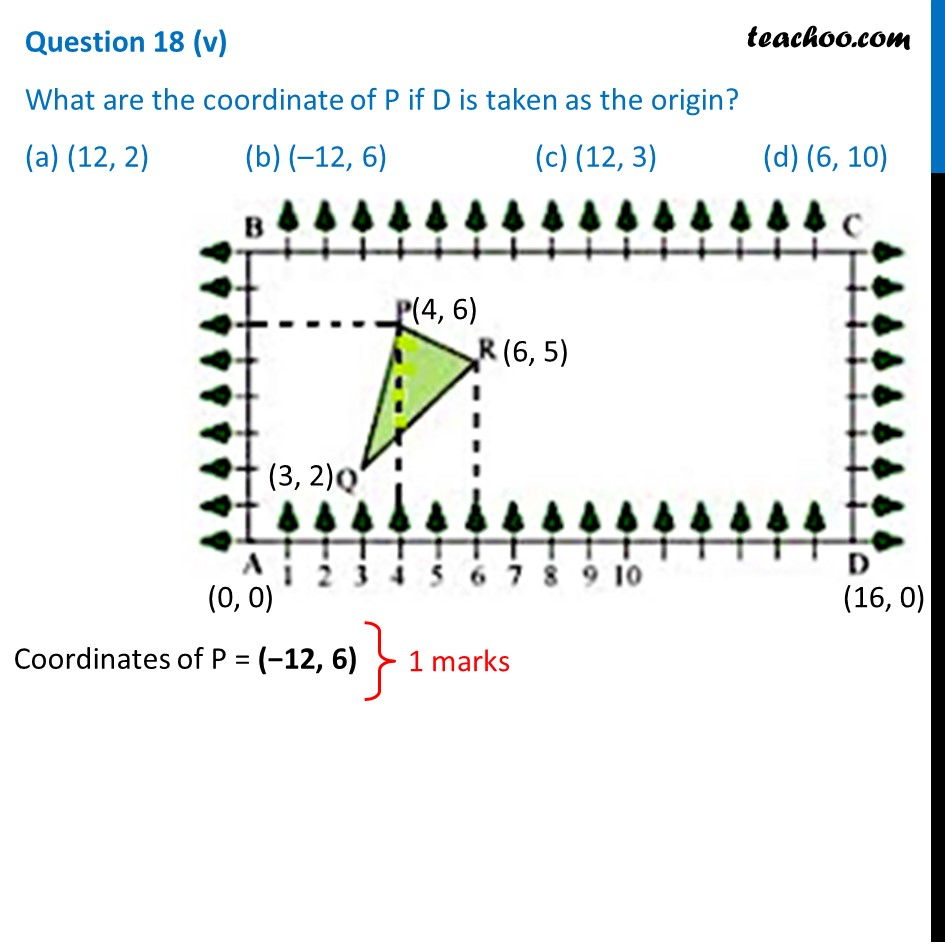Note : This question is inspired from   to Ex 7.4, 5 (Optional) of NCERT – Chapter 7 Class 10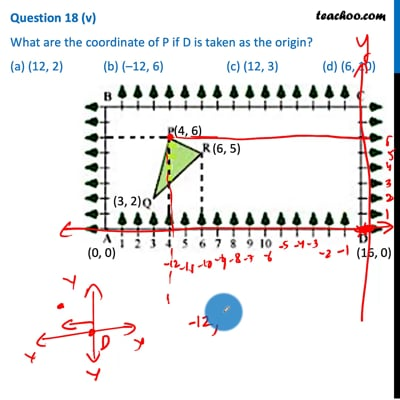This video is only available for Teachoo black users

### Transcript

Question 18 Class X students of a secondary school in Krishnagar have been allotted a rectangular plot of a land for gardening activity. Saplings of Gulmohar are planted on the boundary at a distance of 1m from each other. There is a triangular grassy lawn in the plot as shown in the fig. The students are to sow seeds of flowering plants on the remaining area of the plot. Considering A as origin, answer question (i) to (v)Question 18 (i) Considering A as the origin, what are the coordinates of A? (a) (0, 1) (b) (1, 0) (c) (0, 0) (d) (–1, –1)Coordinates of A = (0, 0) Question 18 (ii) What are the coordinates of P? (a) (4, 6) (b) (6, 4) (c) (4, 5) (d) (5, 4)Coordinates of P = (4, 6) Question 18 (iii) What are the coordinates of R? (a) (6, 5) (b) (5, 6) (c) (6, 0) (d) (7, 4) Coordinates of R = (6, 5) Question 18 (iv) What are the coordinates of D? (a) (16, 0) (b) (0, 0) (c) (0, 16) (d) (16, 1) Coordinates of D = (16, 0) Question 18 (v) What are the coordinate of P if D is taken as the origin? (a) (12, 2) (b) (–12, 6) (c) (12, 3) (d) (6, 10) Coordinates of P = (−12, 6)